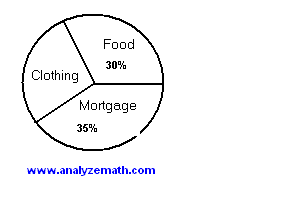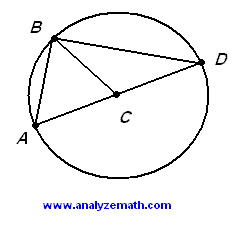# Free SAT Maths Test Practice Questions with Answers Sample 4

A set of Maths questions to practice for the SAT maths test are presented, with detailed solutions and explanations. The answers are at the bottom of the page. A sample of Student-Produced Response Questions are also included.

## A) Multiple Choice Questions

1. Which of the labeled points in the figure below is at a distance of 10 units from the origin of the rectangular coordinate system of axes?.

A) M
B) N
C) P
D) Q
E) R

2. If y = kx + 2k, where k is a constant, and if y = -14 when x = 5, what is the value of x when y = -24?
A) -10
B) 10
C) -24
D) -2
E) 4

3. m and n are integers greater then zero such that 3m = 91/n. What is the value of m*n?
A) 1
B) 3
C) 9
D) 1/2
E) 2

4. If f is a function such that f(x+1) = 2x - 1, then f(2x) =
A) 4x - 1
B) 2x - 2
C) 4x - 4
D) 4x - 3
E) 2

5. A large rectangle is made up of 4 congruent smaller rectangles. What is the length of a small rectangle if the area of the larger rectangle is equal to 432 square units?.

A) 18
B) 108
C) 27
D) 71
E) 54

6. The result of 2 + 25% + 1.6 is equivalent to
A) 3.625
B) 3.25
C) 3(1/4) (mixed number)
D) 385%
E) 28.6%

7. Which of these points is inside the closed curve defined by the equation (x - 2)2 + (y + 3)2 = 4?
A) (0 , -3)
B) (3 , -2)
C) (4 , 1)
D) (1 , 4)
E) (0 , 0)

8. The perimeter of a rectangular field is 8 times its width. The area of the field is 48 meters squared. What is the perimeter, in meters, of the field?
A) 6
B) 48
C) 8
D) 16
E) 32

9. The area of a triangle with side lengths 2.4, 1.8 and 3.0 is equal to
A) 7.2
B) 2.16
C) 2.7
D) 3.6
E) 4.32

10. The number of books N to be sold at a book fare is given by the function
N(p) = 25000 / (3p + k)

where p is the price per book and k is a constant. If 1000 books are sold at $7 per book, how many books will be sold at the price of$10 per book?
A) 735
B) 1429
C) 1430
D) 730
E) 1431

11. For what value(s) of the parameter m does the equation
-2x2 + mx = 2

have one solution only?
A) 0
B) -2 , 2
C) -1 , 1
D) 16
E) -4 , 4

12. The average of the first 4 data values of a data set is equal to 21. The average of the last two data values of the set is equal to 27. What is the average of the 6 data values?
A) 24
B) 22
C) 23
D) 20
E) 21

13. If the values of the 4th and 7th terms of an arithmetic sequence are 6.5 and 11, then the value of the 20th term is
A) 32
B) 31
C) 31.5
D) 30.5
E) 30

14. A store is offering a 15% reduction of all regular prices such that the new price of a flat screen TV set is $680. What was the price, in dollars, of the TV set before the reduction? A) 591 B) 800 C) 665 D) 780 E) 900 15. (xy)4n - (xy)2n is equivalent to A) (xy)6n B) (xy)2n C) [ (xy)2n - (xy)n ] [(xy)2n + (xy)n ] D) [ (xy)2n - (xy)n ] [(xy)2n - (xy)n ] E) [ (xy)2n - (xy)n ] 2 16. The pie graph below shows the expenses of the Taylors family in a particular month. If the Taylors spent$600 on food, how much did they spend that month on clothing?.

A) 350
B) 400
C) 500
D) 600
E) 700

17. Which of these is not correct?

A) sqrt[ x2 + 2x + 1 ] = |x + 1|
B) |- x2 - 1| = x2 + 1
C) sqrt(x4) = x2
D) (-x2 - 6x - 9) / (- x - 3) = - x - 3
E) |e - 1/2 - 3| = 3.5 - e

18. Functions f and g are graphed below. what are the values of x between -1 and 5 (inclusive) for which f(x) > g(x)?.

A) [-1 , 5]
B) [-1 , 0) U (0 , 4) U (4 , 5]
C) [-1 , 0) U (4 , 5]
D) [2 , 5]
E) [0 , 4]

19. A circle of center C is shown below. A, B and D are points on the circle such that A, C and D are collinear. If the lengths of the segments AB and BC are equal, then the size of angle BDC is equal to.

A) 10
B) 30
C) 50
D) 60
E) 90

20. Twice the difference of the squares of two consecutive positive integers is 4n. What is the sum of the two integers?
A) 2n
B) 2
C) 4
D) 4n
E) 1

## B) Student-Produced Response Questions

1. Points A(2,2), B(x,y) and C(8,6) are collinear and point B is between points A and C. The length of segment AB is equal to 1/5 the length of segment AC. Find the coordinates of point B.

2. What is the value of x If (x2 + 3)(2|x| + 4)(-x + 3) = 0?

3. n is a positive integer divisible by 3 and 7. What is the reminder when 2(n + 1) + 3 is divided by 42?

4. x and y are numbers such that (1/2)x + (1/5)y = 1/5 and (1/5)x + (1/2) y = 1/5. What is the value of 2(x + y)?

5. Pedro drove for one hour and a half at the rate of 60 kilometers per hour and for 3 hours at the rate of 70 kilometers per hour. What was Pedro's average speed, kilometers per hour, for the whole journey?

6. Find the smallest number that is larger than 1000 and divisible by 3, 11 and 12.

7. Find a positive integer N such that when N is divided by 7 it gives a reminder equal to 3 and when N is divided by 5 the reminder is equal to 1.

8. The difference of the squares of two consecutive positive even integers is 20. What is the average of the two numbers?

9. If x + 2y + 3z = 20 and 3x + 2y - z = 10, what is the value of 5x + 4y?

10. Each one of the 30 students at the cafeteria bought a sandwich only, a drink only or a sandwich and a drink. If 8 students bought a sandwich only and 12 students bought a drink only, how many students bought a sandwich and a drink?

11. If 2/3 of a number is equal to 5/3, what is twice the number?

1. D
2. B
3. E
4. D
5. A
6. D
7. B
8. E
9. B
10. A
11. E
12. C
13. D
14. B
15. C
16. E
17. D
18. C
19. B
20. A

## B) Student-Produced Response Questions

1. (16/5 , 14/5)
2. 3
3. 5
4. 8/7
5. 66.7 (rounded to 1 decimal place)
6. 1056
7. 31
8. 5
9. 25
10. 10
11. 5

More Maths Practice Tests
Free Practice for SAT, ACT Math tests
Free Practice for GAMT Math tests
Free Compass Math tests Practice
Free GRE Quantitative for Practice
Free AP Calculus Questions (AB and BC) with Answers